# Binary Min and Max Curvature Flow of Binary Image#

## Synopsis#

BinaryMinMaxCurvatureFlow a binary image.

## Code#

### C++#

#include "itkImage.h"
#include "itkBinaryMinMaxCurvatureFlowImageFilter.h"
#include "itkSubtractImageFilter.h"

#include "itksys/SystemTools.hxx"

#ifdef ENABLE_QUICKVIEW
#  include "QuickView.h"
#endif

int
main(int argc, char * argv[])
{
if (argc < 2)
{
std::cerr << argv << " InputFileName [NumberOfIterations]" << std ::endl;
return EXIT_FAILURE;
}

std::string inputFileName = argv;

unsigned int numberOfIterations = 2;
if (argc > 2)
{
numberOfIterations = std::stoi(argv);
}

constexpr unsigned int Dimension = 2;

using InputPixelType = float;
using OutputPixelType = float;
using InputImageType = itk::Image<InputPixelType, Dimension>;
using OutputImageType = itk::Image<OutputPixelType, Dimension>;

using FilterType = itk::BinaryMinMaxCurvatureFlowImageFilter<InputImageType, OutputImageType>;
auto filter = FilterType::New();
filter->SetInput(input);
filter->SetThreshold(255);
filter->SetNumberOfIterations(numberOfIterations);

using SubtractType = itk::SubtractImageFilter<OutputImageType>;
auto diff = SubtractType::New();
diff->SetInput1(input);
diff->SetInput2(filter->GetOutput());

#ifdef ENABLE_QUICKVIEW
QuickView viewer;

std::stringstream desc;
desc << "BinaryMinMaxCurvature, iterations = " << numberOfIterations;

std::stringstream desc2;
desc2 << "Original - BinaryMinMaxCurvatureFlow";

viewer.Visualize();
#endif

return EXIT_SUCCESS;
}


## Classes demonstrated#

template<typename TInputImage, typename TOutputImage>
class BinaryMinMaxCurvatureFlowImageFilter : public itk::MinMaxCurvatureFlowImageFilter<TInputImage, TOutputImage>

Denoise a binary image using min/max curvature flow.

BinaryMinMaxCurvatureFlowImageFilter implements a curvature driven image denoising algorithm. This filter assumes that the image is essentially binary: consisting of two classes. Iso-brightness contours in the input image are viewed as a level set. The level set is then evolved using a curvature-based speed function: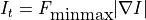where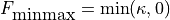if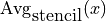is less than or equal to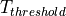and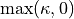, otherwise.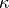is the mean curvature of the iso-brightness contour at point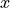.

In min/max curvature flow, movement is turned on or off depending on the scale of the noise one wants to remove. Switching depends on the average image value of a region of radius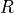around each point. The choice of, the stencil radius, governs the scale of the noise to be removed.

The threshold valueis a user specified value which discriminates between the two pixel classes.

This filter make use of the multi-threaded finite difference solver hierarchy. Updates are computed using a BinaryMinMaxCurvatureFlowFunction object. A zero flux Neumann boundary condition is used when computing derivatives near the data boundary.

Reference: “Level Set Methods and Fast Marching Methods”, J.A. Sethian, Cambridge Press, Chapter 16, Second edition, 1999.

Warning

This filter assumes that the input and output types have the same dimensions. This filter also requires that the output image pixels are of a real type. This filter works for any dimensional images.

See

BinaryMinMaxCurvatureFlowFunction

See

CurvatureFlowImageFilter

See

MinMaxCurvatureFlowImageFilter

ITK Sphinx Examples: